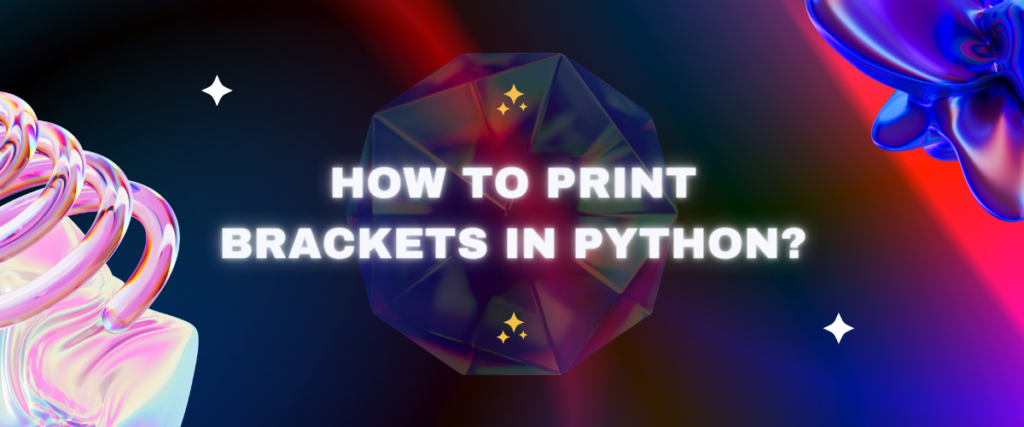# How to Print Brackets in Python?Python has very simple syntaxes. Python as a language is beginner friendly. A programmer who is new to this field may get afraid of programming due to all these complex syntaxes in languages like C and Java. But Python also brings some complexities with its short and simple syntaxes. One of these issues is printing brackets.

In this article, we’re going to check how we can print brackets in Python.

## What do brackets signify in Python?

Brackets in almost all programming languages are metacharacters. That means they are used to specify something. In Python, we use indentation instead of curly braces, but curly braces are still used just not for indentation. They are used for denoting a set or a dictionary. Square brackets are used to denote a list or used for indexing in indexed iterables like lists and tuples. Parenthesis is used to specify the order of operations and in calling a function or creating an instance of a class, or it denotes a tuple.

## Printing Parentheses

Parentheses are “()”. They are used to call a function or create a tuple. The `print` function itself uses parenthesis. Let’s see how you can print parentheses.

Related: What are tuples in Python?

### Using `print()` function.

`print()` function is the standard function used for printing strings and anything in Python. It is very simple to use. Just type `print("<String you want to print>")`.

```print("(")
print(")")
print("()")
```

The first line of the block of code prints an opening parentheses “(“. The second line prints a closing parentheses “)” and so on the third line prints a paired parentheses “()”.

### Using string concatenation

String concatenation is adding strings instead of numbers. When we concatenate 2 strings, both strings combine and form a single string.

```<str1>+<str2>=<str1><str2>
```

Now let’s see how we can print brackets by concatenating strings.

```empty = ""
print(empty+"(")
print(empty+")")
print(empty+"()")
```

Here, we used an empty string variable to print the brackets. We concatenated the bracket with the empty string and printed it. Here instead of an empty string, you can use any string.

```opening_parentheses = "this is openning parentheses"
print("("+opening_parentheses)
closing_parentheses = "this is closing parentheses"
print(closing_parentheses+")")
parentheses = "This is parentheses"
print("("+parentheses+")")
```

### Using formatted strings

A format string can be created using the `format()` method. In a format string, curly braces are used to represent a dynamic value. We can also use formatted strings to print parentheses. Let’s see how we can do it.

```print("Parentheses: {}hello{}".format("(",")"))
```

Output:
`Parentheses: (hello)`

We used the format() method to print parentheses in the above code. We placed curly braces where we wanted our brackets, and in the `format()` function, replaced {} with () or parentheses.

## Printing Square Brackets

Square brackets are used to create lists. Let’s see how we can print square brackets in Python.

Related: What are lists in Python?

### Using `print()` function.

```print("[")
print("]")
print("[]")
```

The first line of the block of code prints an opening square bracket “[“. The second line prints a closing square bracket “]” and so on the third line prints a paired square bracket “[]”.

### Using string concatenation

Now let’s see how we can print square brackets by concatenating strings.

```empty = ""
print(empty+"[")
print(empty+"]")
print(empty+"[]")
```

Here, we used an empty string variable to print the brackets. We concatenated the bracket with the empty string and printed it. Here instead of an empty string, you can use any string.

```opening_bracket = "this is an opening square bracket"
print("["+opening_bracket)
closing_bracket = "this is closing square bracket"
print(closing_bracket+"]")
square_brackets = "This is a square bracket"
print("["+square brackets+"]")
```

### Using formatted strings

We can also use formatted strings to print square brackets. Let’s see how we can do it.

```print("Square brackets: {}hello{}".format("[","]"))
```

Output:
`Square brackets: [hello]`

We used the format() method to print square brackets in the above code. We placed curly braces where we wanted our brackets and in the `format()` function, replaced {} with [] or square brackets.

## Printing Curly Braces

Curly braces are used to create a dictionary and sets. They are also used to get dynamic values in formatted strings. Let’s see how we can print curly braces in Python.

Related: What is a dictionary in Python?

### Using `print()` function.

```print("{")
print("}")
print("{}")
```

The first line of the block of code prints an opening curly brace “{“. The second line prints a closing curly brace “}” and so on the third line prints paired curly braces “{}”.

### Using string concatenation

Now let’s see how we can print curly braces by concatenating strings.

```empty = ""
print(empty+"{")
print(empty+"}")
print(empty+"{}")
```

Here, we used an empty string variable to print the braces. We concatenated the curly braces with the empty string and printed it. Here instead of an empty string, you can use any string.

```opening_brace = "this is an opening curly brace"
print("["+opening_brace)
closing_brace = "this is closing square brace"
print(closing_brace+"]")
curly_braces = "This is curly braces"
print("{"+curly braces+"}")
```

### Using formatted strings

We can also use formatted strings to print curly braces. Let’s see how can we do it.

```print("Curly braces: {}hello{}".format("{","}"))
```

Output:
`Curly Braces: {hello}`

We used the format() method to print curly. braces in the above code. We placed curly braces where we wanted our braces and in the `format()` function, replaced {} with {} or curly braces.

## Conclusion

That was it for this article. It was a simple but interesting question. It’s good to keep asking questions. This is what makes us good programmers. Another essential part of programming is practice. Practice is never enough; the more you practice, the better you get at programming.

## References

Stack Overflow answer for the same question.